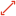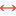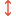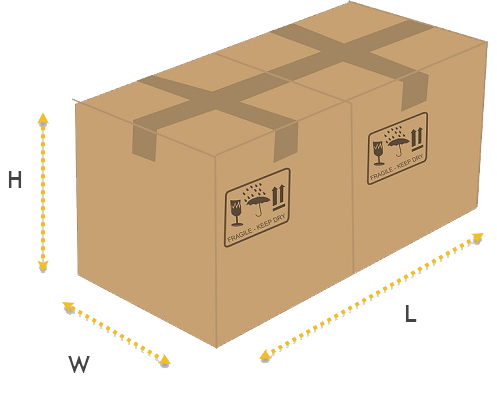# Dimensional Weight

It means that the received package is big, but relatively light. It may be a good idea to ship items separately. «Dimensional weight» means that actual weight is two (or more) times smaller than dimensional weight# Dimensional Weight Calculator:Dimensional weight= 7.2lb (7.2kg)

Example: when actual weight of package is 10lb. but dimensional is > 150lb we consider the package as having heavy dimension weight.

# Actual Weight

Actual weight is the weight of the package when we put it on our scales.# Dimensional weight

Dimensional weight is based on the size of the package. Dimensional weight is calculated by using the formula: